# Additive divisor problem

Jump to: navigation, search

The problem of finding asymptotic values for sums of the form:(1)

whereis the number of different factorizations of an integerinfactors, counted according to multiplicity. Here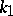and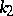are integers,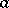is a fixed integer different from zero andis a sufficiently large number. In particular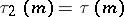is the number of divisors of the number. Sums of the form (1) express the number of solutions of the equations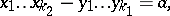(2)(3)

respectively. Particular cases of the additive divisor problem (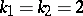,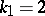and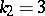) are considered in . The additive divisor problem withand an arbitrary positive integerwas solved using the dispersion method of Yu.V. Linnik .